#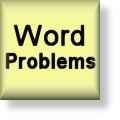Word Problems Worksheets

## Dynamically Created Word Problems

Here is a graphic preview for all of the word problems worksheets. You can select different variables to customize these word problems worksheets for your needs. The word problems worksheets are randomly created and will never repeat so you have an endless supply of quality word problems worksheets to use in the classroom or at home. Our word problems worksheets are free to download, easy to use, and very flexible.

These word problems worksheets are a great resource for children in 3rd Grade, 4th Grade, and 5th Grade.

Click here for a Detailed Description of all the Word Problems Worksheets.

## Quick Link for All Word Problems Worksheets

Click the image to be taken to that Word Problems Worksheet.

##### Words to SymbolsWord Problems##### Addition Word ProblemsUsing 1 Digit with 2 Addends##### Addition Word ProblemsUsing 2 Digit with 2 Addends##### Addition Word ProblemsUsing 1 Digit with 3 Addends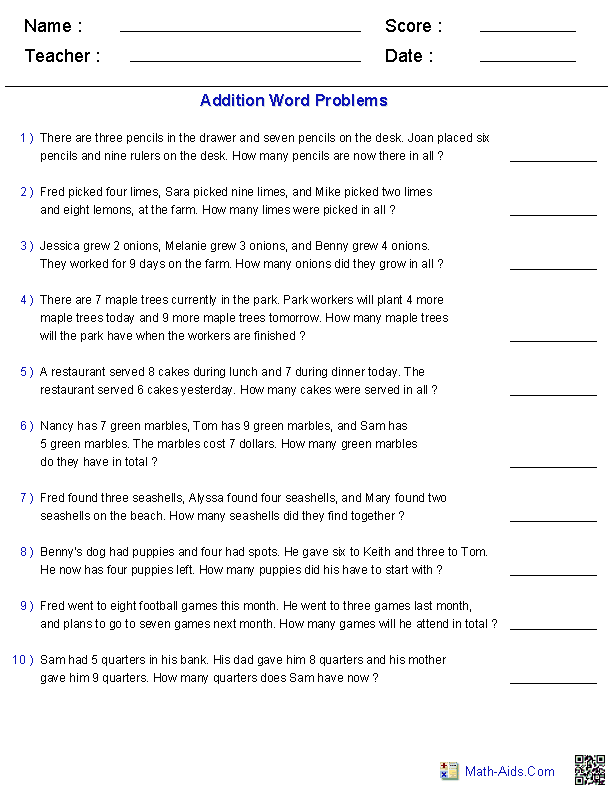##### Addition Word ProblemsUsing 2 Digit with 3 Addends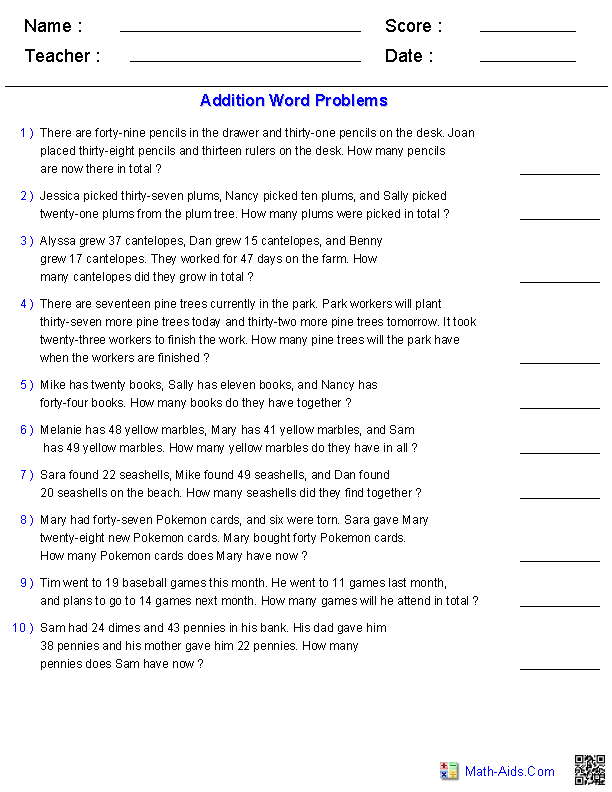##### Addition Word Problems2 Digit Missing Addends##### Subtraction Word ProblemsUsing 1 Digit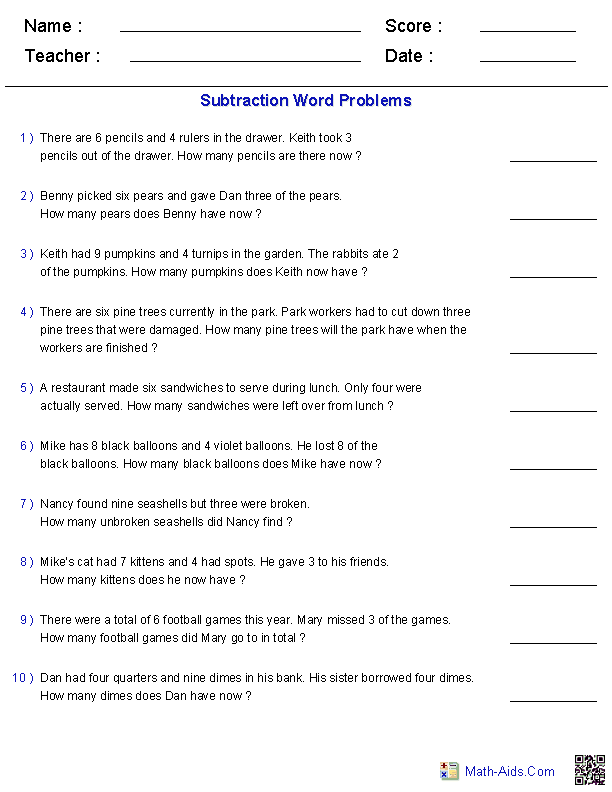##### Subtraction Word ProblemsUsing 2 Digits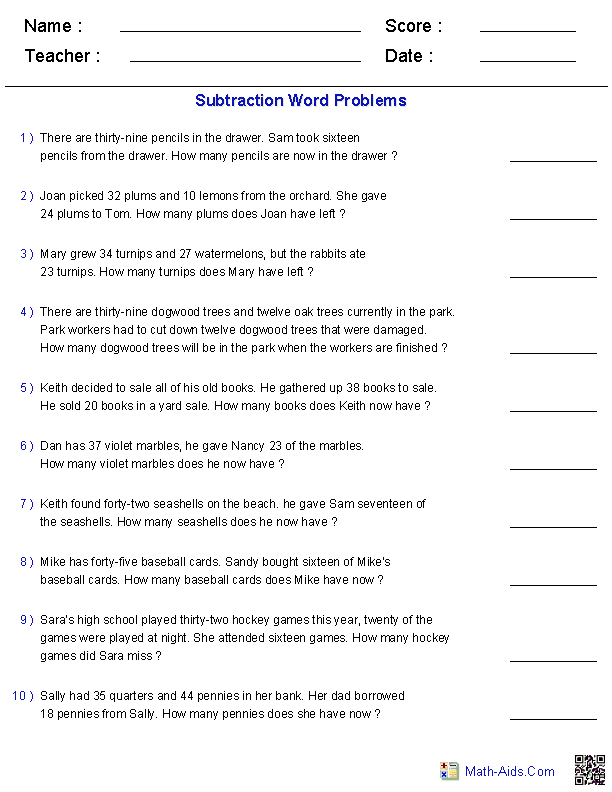##### Addition and SubtractionWord Problems Using 1 Digit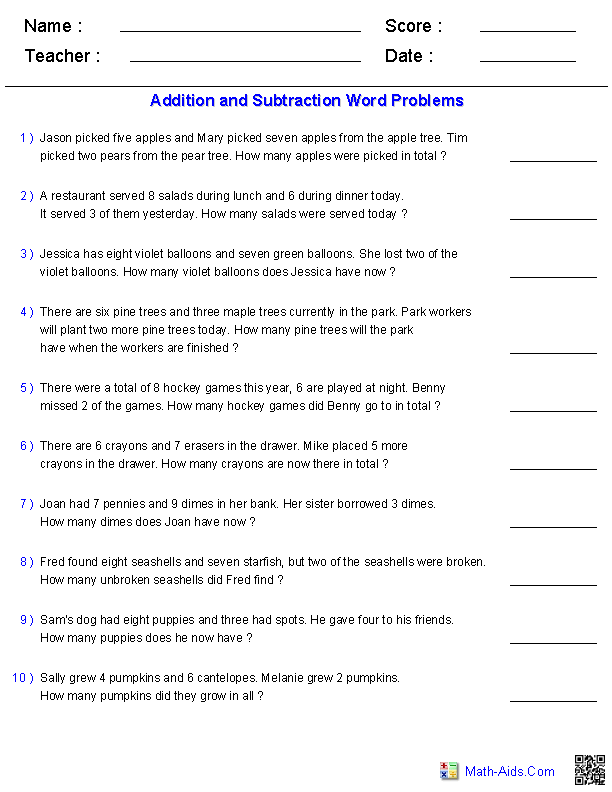##### Addition and SubtractionWord ProblemsUsing 2 Digits##### Addition and SubtractionWord Problems 2 DigitsWith No Regrouping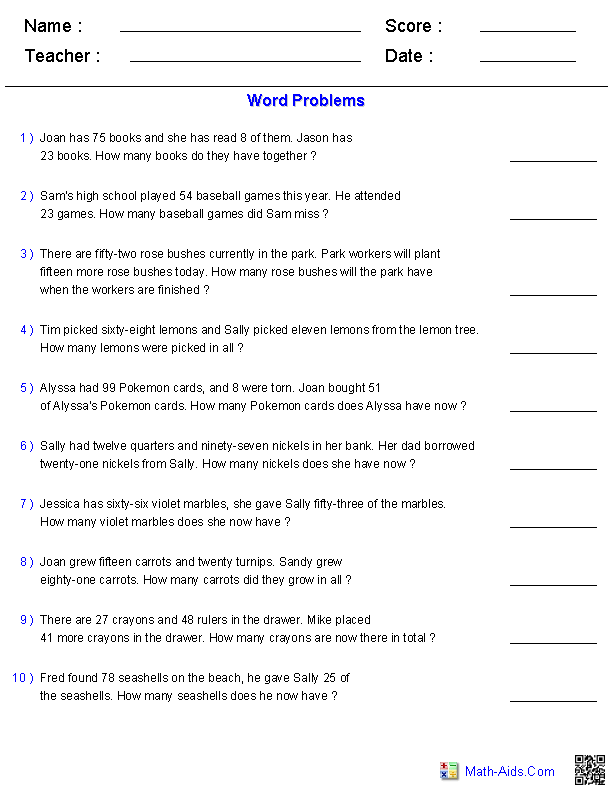##### Addition and SubtractionWord ProblemsUsing 3 Digits##### Multi-Step ProblemsAddition and Subtraction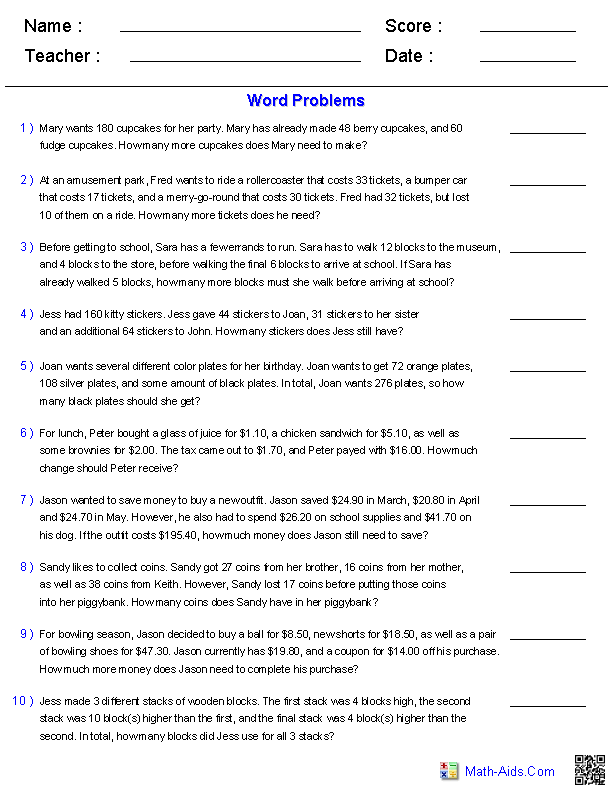##### Multiplication Word ProblemsUsing 1 Digit##### Multiplication Word ProblemsUsing Dozens##### Multiplication Word ProblemsUsing 2 Digits##### Division Word ProblemsUsing 1 Digit in Divisor##### Division Word ProblemsUsing Dozens in Divisor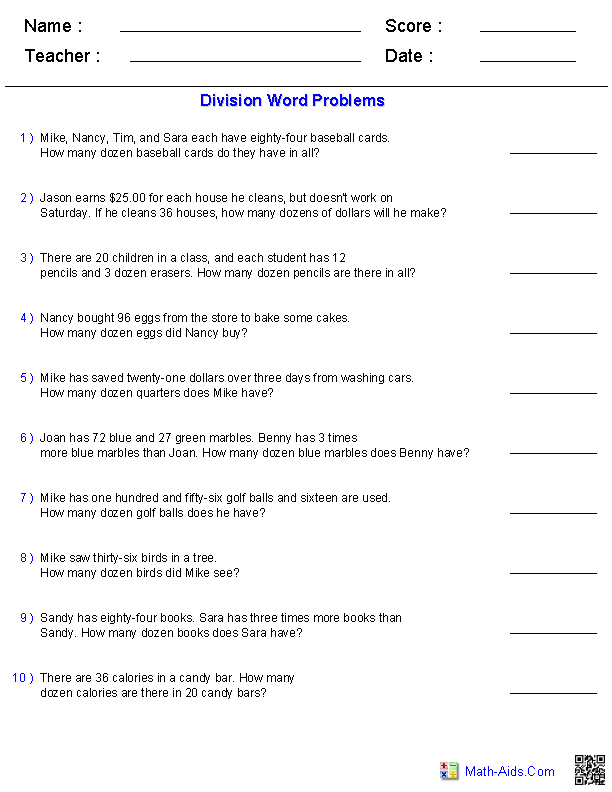##### Division Word ProblemsUsing Multiple Digits in Divisor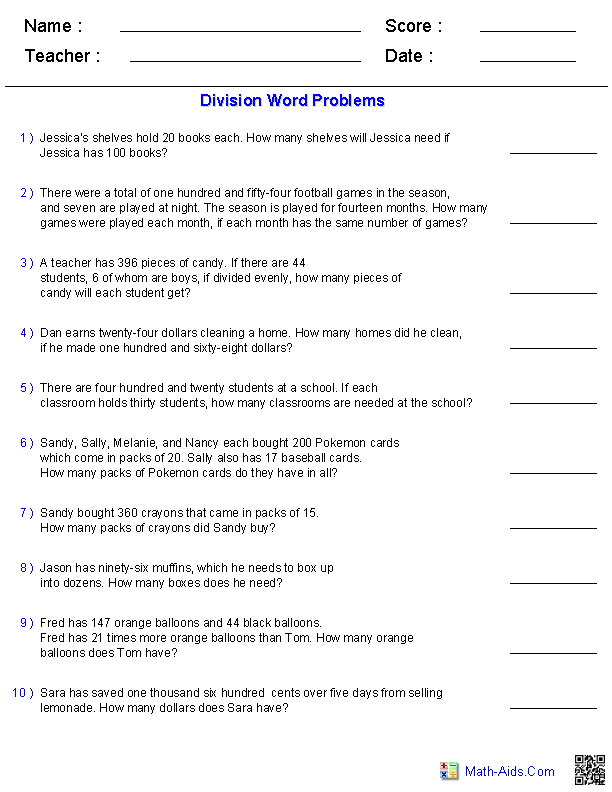##### Division Word ProblemsDividing with Fractions##### Multiplication and DivisionWord Problems Using 1 Digit##### Mixed Operations ProblemsUsing 1 or 2 Digits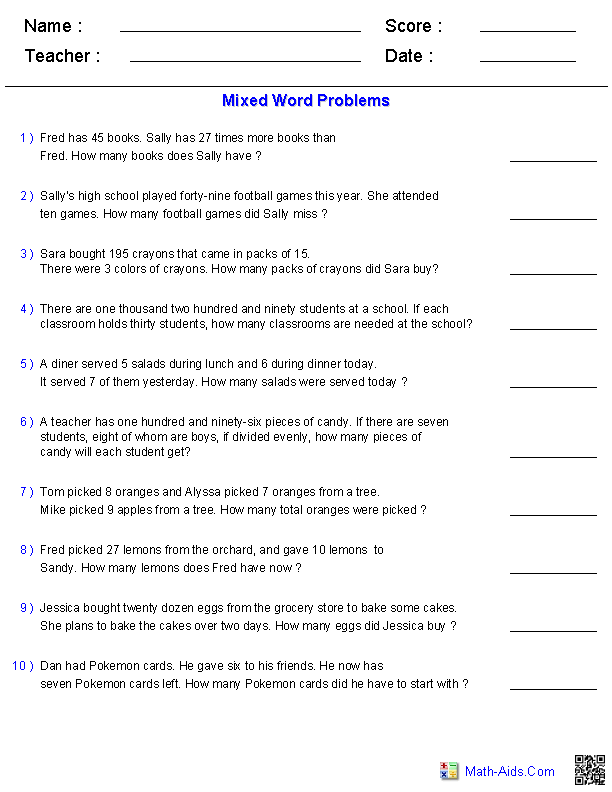##### One Step EquationWord Problems##### Two Step EquationWord Problems##### Multi-Step All OperationsWord Problems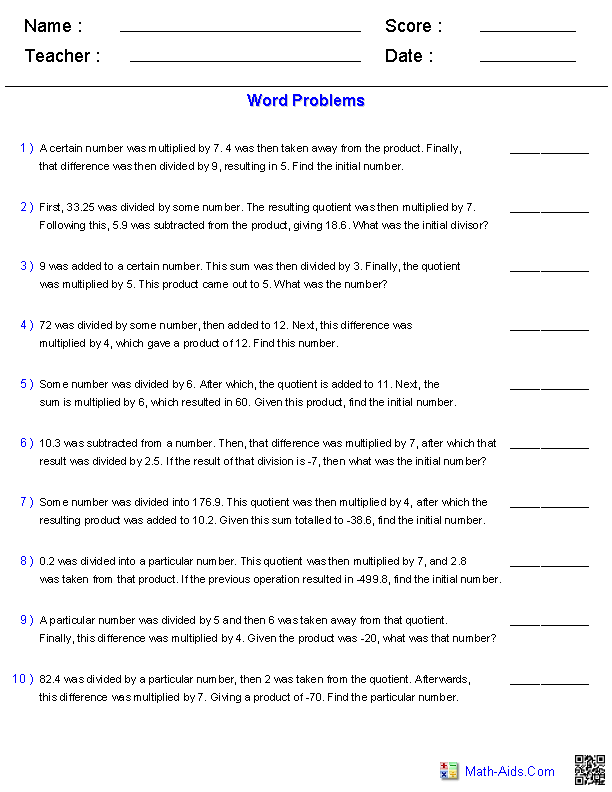##### Fractions Word ProblemsAdding Two Fractions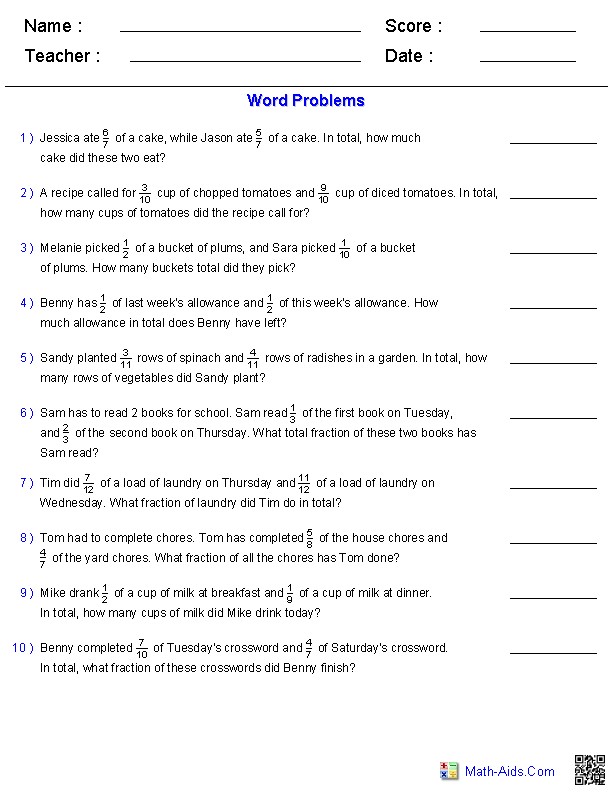##### Fractions Word ProblemsAdding Three Fractions##### Fractions Word ProblemsSubtracting Fractions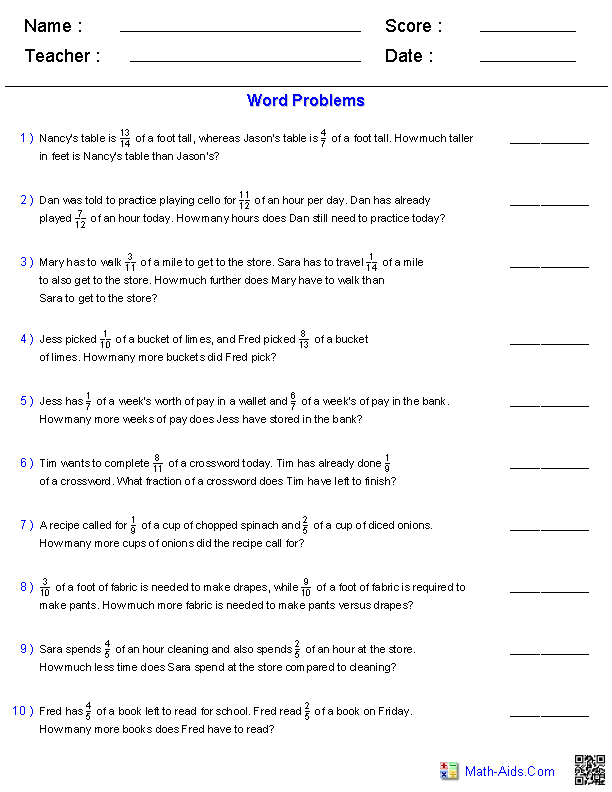##### Fractions Word ProblemsAdding Two Mixed Numbers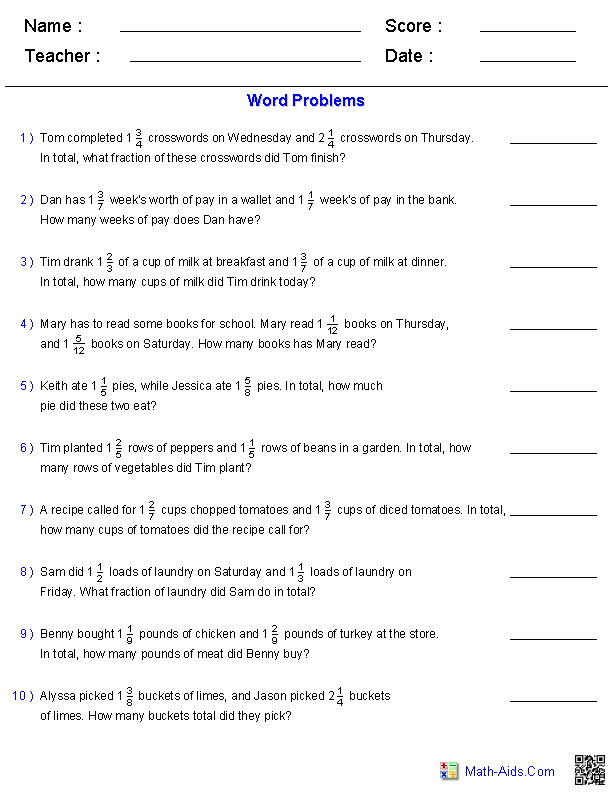##### Fractions Word ProblemsSubtracting Two Mixed Numbers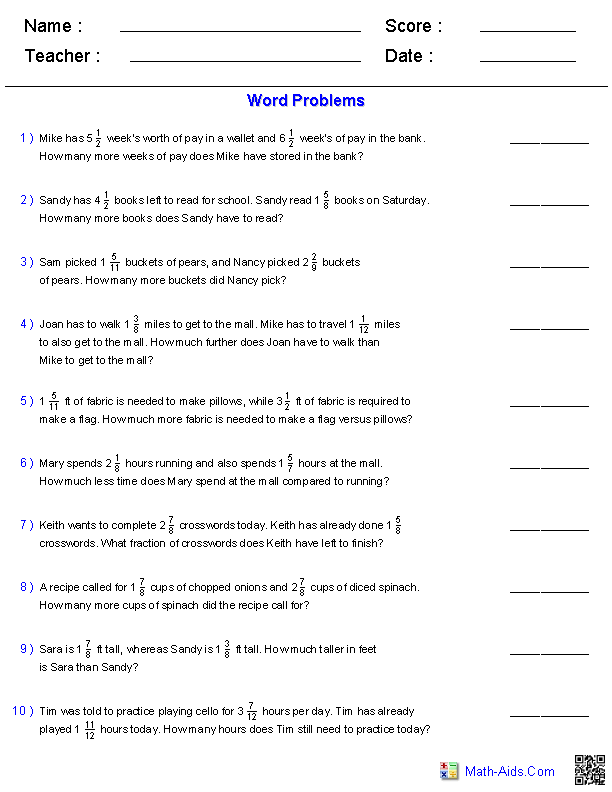##### U. S. Coins Word ProblemsAdding Coins Worksheets##### Advanced Adding U. S. CoinsWord Problems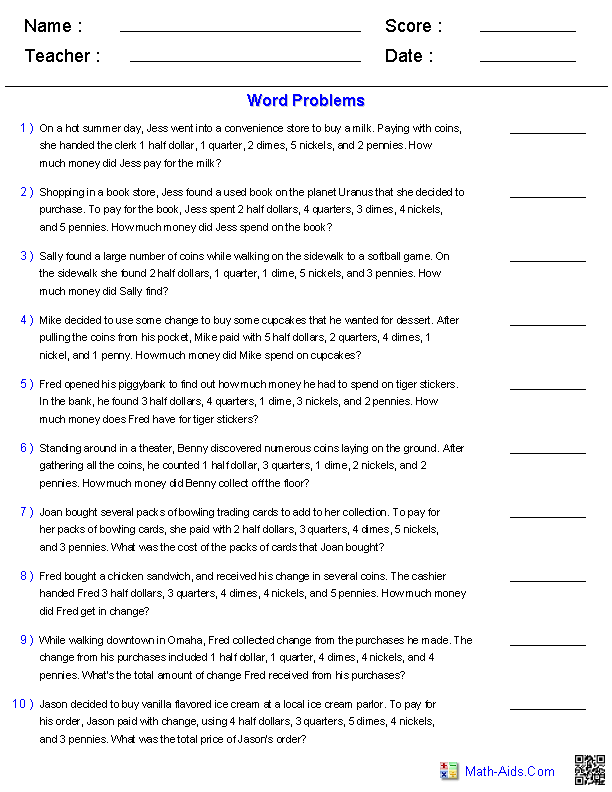##### U. S. Money Word ProblemsAdding Two Purchases##### U. S. Money Word ProblemsAdding Three Purchases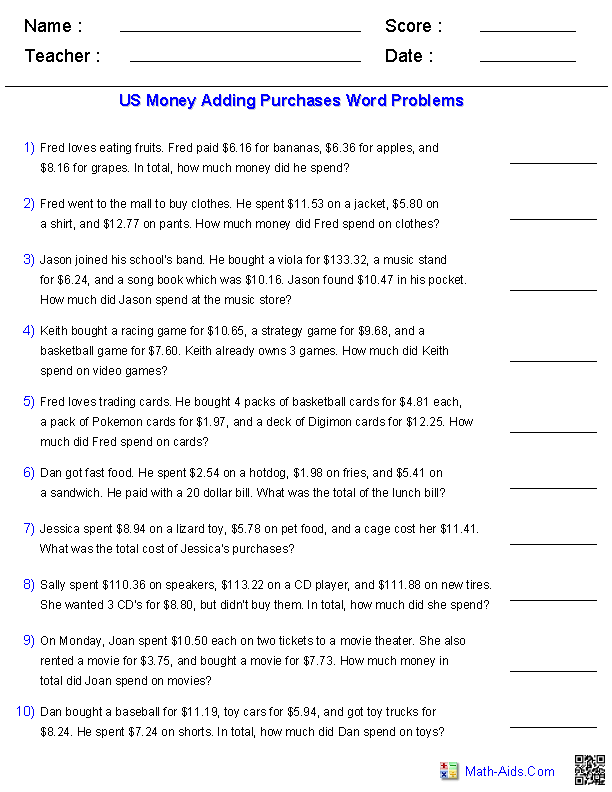##### U. S. Money Word ProblemsChange from a Purchase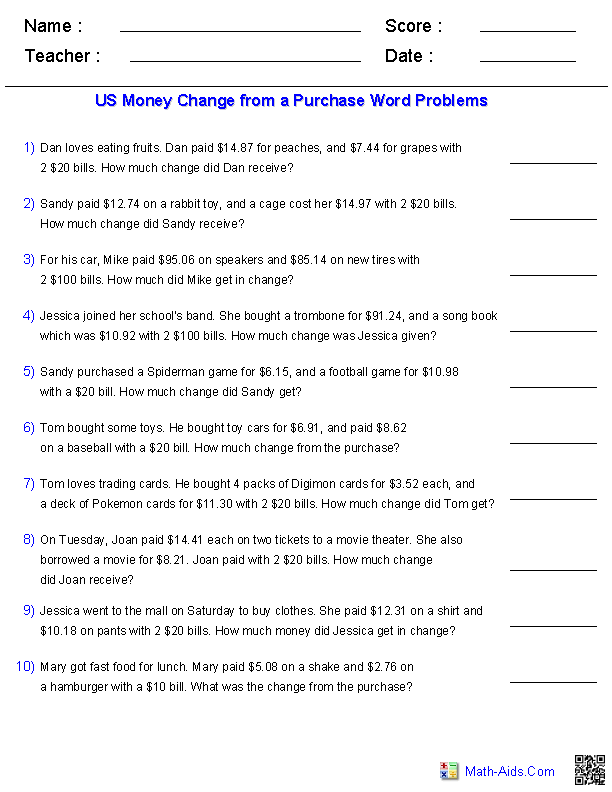##### Travel TimeWord Problems##### Reading a CalendarWord Problems##### Elapsed DatesWord Problems##### Ratios and RateWord Problems##### PercentageWord Problems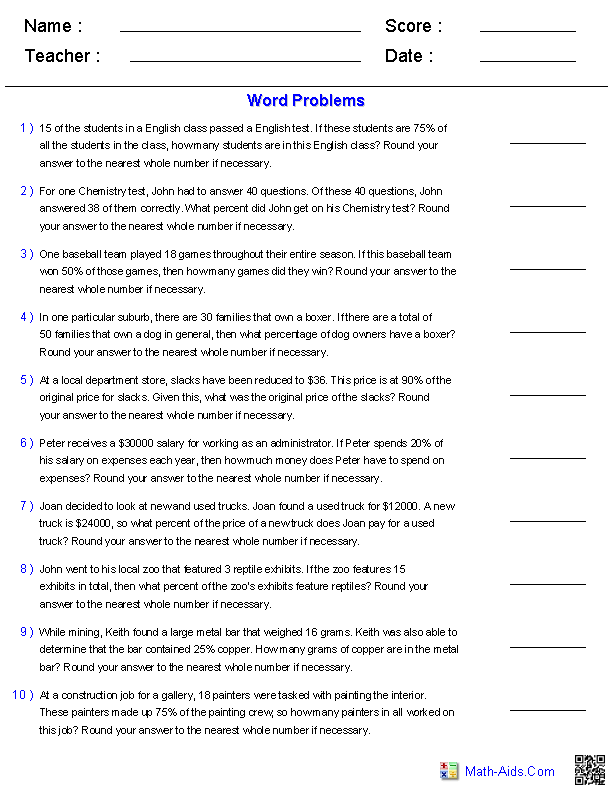##### Mixed Operationswith Key PhrasesWord Problems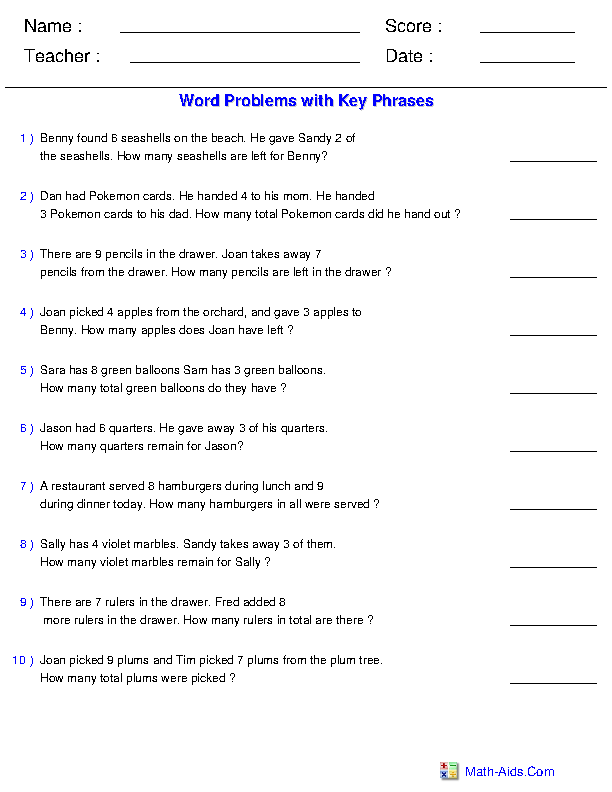##### U.S. Money Change from a Purchase Multiplication Word Problems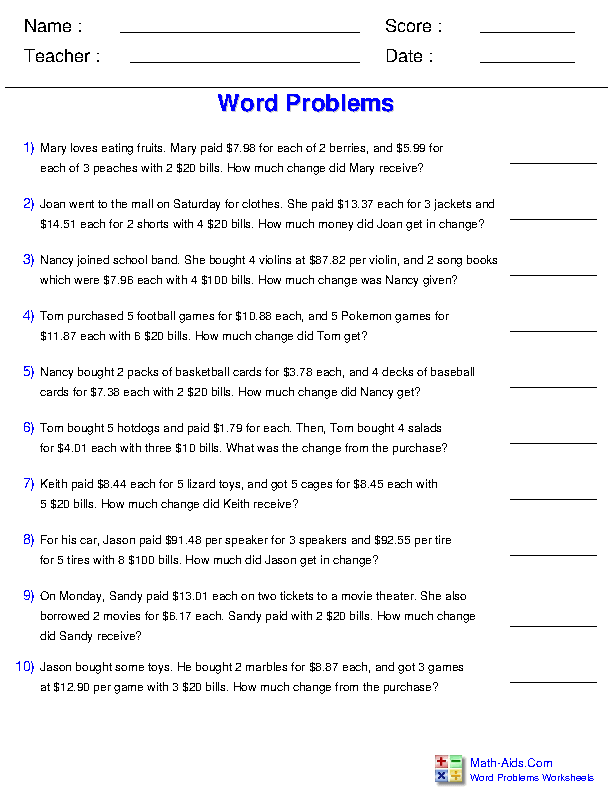Recommended Videos

## Detailed Description for All Word Problems Worksheets

Words to Symbols Handout
This Word Problems Worksheet will produce a great handout to help students learn the symbols for different words and phrases in word problems.

Addition Word Problems Worksheets Using 1 Digit with 2 Addends
These addition word problems worksheets will produce 1 digit problems with two addends, with ten problems per worksheet. These word problems worksheets are appropriate for 3rd Grade, 4th Grade, and 5th Grade.

Addition Word Problems Worksheets Using 2 Digits with 2 Addends
These addition word problems worksheets will produce 2 digits problems with two addends, with ten problems per worksheet. These word problems worksheets are appropriate for 3rd Grade, 4th Grade, and 5th Grade.

Addition Word Problems Worksheets Using 1 Digit with 3 Addends
These addition word problems worksheets will produce 1 digit problems with three addends, with ten problems per worksheet. These word problems worksheets are appropriate for 3rd Grade, 4th Grade, and 5th Grade.

Addition Word Problems Worksheets Using 2 Digits with 3 Addends
These addition word problems worksheets will produce 2 digits problems with three addends, with ten problems per worksheet. These word problems worksheets are appropriate for 3rd Grade, 4th Grade, and 5th Grade.

Addition Word Problems Worksheets 2 Digits Missing Addends
These addition word problems worksheet will produce 2 digits problems with missing addends, with ten problems per worksheet. You may select between regrouping and non-regrouping type of problems. These word problems worksheets are appropriate for 3rd Grade, 4th Grade, and 5th Grade.

Subtraction Word Problems Worksheets Using 1 Digit
These subtraction word problems worksheets will produce 1 digit problems, with ten problems per worksheet. These word problems worksheets are appropriate for 3rd Grade, 4th Grade, and 5th Grade.

Subtraction Word Problems Worksheets Using 2 Digits
These subtraction word problems worksheets will produce 2 digits problems, with ten problems per worksheet. These word problems worksheets are appropriate for 3rd Grade, 4th Grade, and 5th Grade.

Addition and Subtraction Word Problems Worksheets Using 1 Digit
These addition and subtraction word problems worksheets will produce 1 digit problems, with ten problems per worksheet. These word problems worksheets are appropriate for 3rd Grade, 4th Grade, and 5th Grade.

Addition and Subtraction Word Problems Worksheets Using 2 Digits
These addition and subtraction word problems worksheets will produce 2 digits problems, with ten problems per worksheet. These word problems worksheets are appropriate for 3rd Grade, 4th Grade, and 5th Grade.

Addition and Subtraction Word Problems Worksheets 2 Digits With No Regrouping
These addition and subtraction word problems worksheets will produce 2 digits problems with no regrouping and ten problems per worksheet. These word problems worksheets are appropriate for 3rd Grade, 4th Grade, and 5th Grade.

Addition and Subtraction Word Problems Worksheets Using 3 Digits
These addition and subtraction word problems worksheets will produce 3 digits problems, with ten problems per worksheet. These word problems worksheets are appropriate for 3rd Grade, 4th Grade, and 5th Grade.

Multi-Step Problems Addition and Subtraction
These multi-step addition and subtraction word problems worksheets will produce 10 problems per worksheet. These word problems worksheets are appropriate for 3rd Grade, 4th Grade, and 5th Grade.

Multiplication Word Problems Worksheets Using 1 Digit
These multiplication word problems worksheets will produce 1 digit problems, with ten problems per worksheet. These word problems worksheets are appropriate for 3rd Grade, 4th Grade, and 5th Grade.

Multiplication Word Problems Worksheets Using Dozens
These multiplication word problems worksheets will produce problems using dozens, with ten problems per worksheet. These word problems worksheets are appropriate for 3rd Grade, 4th Grade, and 5th Grade.

Multiplication Word Problems Worksheets Using 2 Digits
These multiplication word problems worksheets will produce 2 digits problems, with ten problems per worksheet. These word problems worksheets are appropriate for 3rd Grade, 4th Grade, and 5th Grade.

Division Word Problems Worksheets Using 1 Digit in Divisor
These division word problems worksheets will produce 1 digit divisor problems, with ten problems per worksheet. These word problems worksheets are appropriate for 3rd Grade, 4th Grade, and 5th Grade.

Division Word Problems Worksheets Using Dozens in Divisor
These division word problems worksheets will produce problems using dozens in the divisor, with ten problems per worksheet. These word problems worksheets are appropriate for 3rd Grade, 4th Grade, and 5th Grade.

Division Word Problems Worksheets Using Multiple Digits in Divisor
These division word problems worksheets will produce multiple digits in the divisor for the problems, with ten problems per worksheet. These word problems worksheets are appropriate for 3rd Grade, 4th Grade, and 5th Grade.

Dividing with Fractions Worksheets
These division Word Problems Worksheets will produce problems that focus on division with fractions. You have the option to select the range of denominators, as well as the types of fractions displayed. These word problems worksheets are appropriate for 4th Grade, 5th Grade, and 6th Grade.

Multiplication and Division Problems Using 1 Digit
These multiplication and division word problems worksheets will produce 1 digit problems, with ten problems per worksheet. These word problems worksheets are appropriate for 3rd Grade, 4th Grade, and 5th Grade.

Mixed Operations Word Problems Using 1 or 2 Digits
These mixed operations word problems worksheets will produce addition, multiplication, subtraction and division problems with 1 or 2 digit numbers. These word problems worksheets will produce ten problems per worksheet. These word problems worksheets are appropriate for 3rd Grade, 4th Grade, and 5th Grade.

One Step Equation Word Problems
These equations worksheets will produce one step word problems. These worksheets will produce ten problems per worksheet. These word problems worksheets are a good resource for students in the 5th Grade through the 8th Grade.

Two Step Equation Word Problems
These equations worksheets will produce two step word problems. These worksheets will produce ten problems per worksheet. These word problems worksheets are a good resource for students in the 5th Grade through the 8th Grade.

Multi-Step All Operations Word Problems
These Word Problems worksheets will produce word problems involving all basic operations. You may choose the format of the answers. These word problems worksheets will produce ten problems per worksheet. These word problems worksheets are a good resource for students in the 5th Grade through the 8th Grade.

Adding Two Fractions Word Problems
These fractions word problems worksheets will produce problems with the addition of two fractions. These word problems worksheets will produce ten problems per worksheet. These word problems worksheets are a good resource for students in the 5th Grade through the 8th Grade.

Adding Three Fractions Word Problems
These fractions word problems worksheets will produce problems with the addition of three fractions. These word problems worksheets will produce ten problems per worksheet. These word problems worksheets are a good resource for students in the 5th Grade through the 8th Grade.

Subtracting Fractions Word Problems
These fractions word problems worksheets will produce problems involving subtracting two fractions. These word problems worksheets will produce ten problems per worksheet. These word problems worksheets are a good resource for students in the 5th Grade through the 8th Grade.

Adding Two Mixed Numbers Word Problems
These fractions word problems worksheets will produce problems involving adding two mixed numbers. These word problems worksheets will produce ten problems per worksheet. These word problems worksheets are a good resource for students in the 5th Grade through the 8th Grade.

Subtracting Two Mixed Numbers Word Problems
These fractions word problems worksheets will produce problems involving subtracting two mixed numbers. These word problems worksheets will produce ten problems per worksheet. These word problems worksheets are a good resource for students in the 5th Grade through the 8th Grade.

Adding U.S. Coins Word Problems
This U.S. coins word problems worksheet will produce coin addition problems. These worksheets will produce ten problems per worksheet. These word problems worksheets are a good resource for students in the 5th Grade through the 8th Grade.

Advanced Adding U.S. Coins Word Problems
These Word Problems Worksheets will produce word problems that focus on adding up different denominations of US coin currency. You have the option to select any combination of pennies, nickels, dimes, quarters, and half dollars for each worksheet. These worksheets will produce ten problems per worksheet. These word problems worksheets are a good resource for students in the 5th Grade through the 8th Grade.

U.S. Money Adding Two Items Word Problems
These U.S. money word problems worksheest will produce purchase problems for adding two items. These worksheets will produce ten problems per worksheet. These word problems worksheets are a good resource for students in the 5th Grade through the 8th Grade.

U.S. Money Adding Three Items Word Problems
These U.S. money word problems worksheets will produce purchase problems for adding three items. These worksheets will produce ten problems per worksheet. These word problems worksheets are a good resource for students in the 5th Grade through the 8th Grade.

U.S. Money Change from a Purchase Word Problems
These U.S. money word problems worksheets will produce problems for calculating change from a purchase. These word problems worksheets will produce ten problems per worksheet. These word problems worksheets are a good resource for students in the 5th Grade through the 8th Grade.

Travel Time Word Problems Worksheets
These time word problems worksheets produce problems for finding the duration, start, and end times of trips. Users may select the units of time to use in the problems. These word problems worksheets will produce ten problems per worksheet. These word problems worksheets are appropriate for students in the 5th Grade through the 8th Grade.

Reading a Calendar Word Problems Worksheets
These calendar worksheets will produce word problems revolving around reading and understanding a monthly calendar. You may select the month and the year within a range of the years 1800 to 3999. These word problems worksheets will produce nine problems per worksheet. These word problems worksheets are appropriate for students in the 5th Grade through the 8th Grade.

Elapsed Dates Word Problems Worksheets
These time word problems worksheets will produce questions with elapsed days, weeks, months, and years, with ten problems per worksheet. These word problems worksheets are appropriate for 3rd Grade, 4th Grade, and 5th Grade.

Ratios and Rates Word Problems Worksheets
These ratio word problems worksheets will produce eight ratio and rates word problems for the students to solve. These ratio word problems worksheets are appropriate for 3rd Grade, 4th Grade, 5th Grade, 6th Grade, and 7th Grade.

Percentage Word Problems Worksheets
These Percentage Word Problems Worksheets will produce problems that focus on finding and working with percentages. You have the option to select the types of numbers, as well as the types of problem you want. These percentage word problems worksheets are appropriate for 3rd Grade, 4th Grade, 5th Grade, 6th Grade, and 7th Grade.

Mixed Word Problems with Key Phrases Worksheets
These Word Problems Worksheets will produce addition, multiplication, subtraction and division problems using clear key phrases to give the student a clue as to which type of operation to use. These word problems worksheets are appropriate for 4th Grade, 5th Grade, 6th Grade, and 7th Grade.

U.S. Money Change from a Purchase Multiplication Word Problems
These Word Problems Worksheets will produce problems that ask students to use multiplication to calculate the monetary value of a purchase and then find how much change is given from the purchase.

If you need help figuring out how old someone is (or how old you are) given a birthday, you can use this great Age Calculator. to answer those questions and many more!Math-Aids.Com.  All rights reserved. Word Problems Worksheets